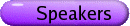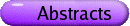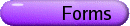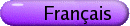Contributed Papers Session
Org: Peter Hoffman (Waterloo)
[PDF]

KATHIE CAMERON, Wilfrid Laurier University
Recent Progress in Colouring Perfect Graphs
[PDF]

Berge defined a graph to be perfect if for every induced subgraph, the minimum number of colours required in a vertex colouring equals the maximum number of vertices in a clique. In 2002, Chudnovsky, Robertson, Seymour and Thomas proved Berge's 40-year-old Strong Perfect Graph Conjecture: a graph is perfect if and only if it contains no odd holes or odd antiholes. A "proof from the book" of this result might be a combinatorial polytime algorithm, which for any graph, finds a clique and colouring the same size, or else finds an odd hole or an odd antihole (or some other easily recognizable combinatorial obstruction to being perfect). In view of precedents, such an algorithm might be simpler than the Chudnovsky-Seymour and Cornuejols-Liu-Vuskovich algorithms for recognizing perfect graphs since it could end up giving a clique and a colouring of the same size in a non-perfect graph. I will report on some recent progress on special classes of graphs in joint work with Jack Edmonds, Elaine Eschen, Chinh Hoang and R. Sritharan.

CRISTIAN ENACHE, Département de mathématiques et de statistique, Université Laval, Cité universitaire, Québec (Québec) G1K 7P4
Spatial decay bounds and continous dependence on the data for the solution of a semilinear heat equation in a long cylindrical region
[PDF]

In this work we study a semilinear heat equation in a long cylindrical region for which the far end and the lateral surface are held at zero temperature and a nonzero temperature is applied at the near end. In other words, the specific domain we consider is a finite cylinder W: = D×[0,L], where D is a bounded convex domain in the (x1,x2)-plane with smooth boundary D Î C2,e, the generators of the cylinder are parallel to the x3-axis and its length is L. The specific problem we consider is the following initial boundary value problem

(1.1)   ì
ï
ï
í
ï
ï
î
 Du-u,t = -f(u),
 x Î W, t Î (0,T),
 u(x,t) = 0,
 x Î ¶WL È ¶Wlat, t Î (0,T),
 u(x,t) = h(x1,x2,t),
 x Î ¶ W0, t Î (0,T),
 u(x,0) = 0,
 x Î W.
(1)
where W0 : = D ×{0}, WL : = D×{L}, Wlat : = D ×(0,L). We also assume that h(x1,x2,t) is a prescribed non-negative function with h(x1,x2,0)=0 and f is a non-negative function satisfying the following conditions
 (1.2)  \undersets® 0 lim f(s) s exists, f¢ (s) £ p(s) and f¢¢ (s) £ q(s) for s ³ 0,
(2)
where p(s) and q(s) are some non-decreasing function of s. We are interested on the spatial decay bounds for the solution of the semilinear heat equation (1.1) and on its continous dependence with respect to the data at the near end of the cylinder. Since the solution u(x,t) of the problem (1.1) can blow up at some point in space time, our aim is to derive sufficient conditions on the data which will guarantee that the solution remains bounded and moreover, under such conditions, we will obtain some explicit spatial decay bounds for the solution, its cross-sectional derivatives and its temporal derivative. We will also prove that the solution depends continously on the data h(x1,x2,t) at the near end of the cylinder.

JOSHUA MACARTHUR, Dalhousie University, Halifax, Nova Scotia B3H 3J5
Computation and Application of the Fundamental Invariants of Vector Spaces of Conformal Killing Vectors
[PDF]

Let C(M) be the vector space of conformal Killing vectors defined on a pseudo-Riemannian manifold M of constant curvature. Consider the action of the isometry group I(M) on C(M). If we employ the method of infinitesimal generators, the problem of finding fundamental invariants and covariants reduces to solving a system of first order linear homogeneous PDEs.

In theory, the method of characteristics may be used to find solutions, however in practice it proves ineffective due to the sheer size of the system. Alternatively, if the invariants or covariants may be represented by polynomials, the problem reduces further to solving a system of linear equations.

The successful application of this alternative to find invariants for all such C(M) in dimensions 3, 4 and 5 will be discussed. In addition, the cases where these invariants have been used to distinguish between equivalence classes in certain C(M) will be shown.

MARNI MISHNA, The Fields Institute for Mathematical Research
Holonomic sequences and walks in the quarter plane
[PDF]

In this talk we will consider the nature of the generating series for different families of walks in the quarter plane. In particular, we consider combinatorial criteria which ensure the holonomy of the counting sequences, that is, when sequences satisfying linear recurrences with polynomial coefficients. We will also ponder the nature of series associated to different classes of formal languages.

Work in collaboration with Mireille Bousquet-Melou and Mike Zabrocki.

MARCO POLLANEN, Trent University, Peterborough, ON K9J 7B8
Algebraic Curves for Non-Uniform Pseudo-Random Sequence Generation
[PDF]

Pseudo-random numbers are a critical part of modern computing, especially for use in simulations and cryptography, and consequently there are a myriad of algorithms for creating uniform pseudo-random sequences. However, many simulations ultimately require non-uniform random sequences. In this talk we introduce a new method to directly generate, without transformation of rejection, some non-uniform pseudo-random sequences. This method is a group-theoretic analogue of linear congruential pseudo-random number generation. We provide examples of such sequences, involving computations in Jacobian groups of plane algebraic curves, that have both good theoretical and statistical properties.

CHRISTIAN ROETTGER, Iowa State University, Math Dept., 472 Carver Hall, Ames, IA 50011, USA
Periodic points classify some families of Markov shifts
[PDF]

Consider the space XG of doubly-indexed sequences over a finite abelian group G satisfying

 xs,t+1 = xs,t + xs+1,t
for all integers s,t. The left and downward shift induce an action of Z2 on XG. Recently, we could prove a conjecture by Ward stating that the periodic point data of XG determine the group G up to isomorphism. Our approach is to view XG as the set of sequences annihilated by T-(S+1) where S,T stand for the two shift actions, and to study algebraic sets of sequences via their annihilators in the polynomial ring Z[S,T]. We will sketch a proof of this theorem and show that our method extends to many other spaces defined by linear recurrences over groups. Key words are Galois Rings, Teichmuller systems and Wieferich primes. The p-adic representation of binomial and multinomial coefficients comes into play.

NAHID SULTANA, Kobe University, Department of Mathematics, 1-1, Rokkodai, Nada-ku, Kobe 657-8501, Japan
Explicit conformal parametrization of Delaunay surfaces in space forms
[PDF]

"Delaunay surfaces" are translationally-periodic constant mean curvature (CMC) surfaces of revolution. We compute explicit conformal parametrizations of Delaunay surfaces in each of the three space forms Euclidean 3-space R3, spherical 3-space S3 and hyperbolic 3-space H3 by using the generalized Weierstrass type representation for CMC surfaces established by J. Dorfmeister, F. Pedit and H. Wu. This method is commonly called the DPW method, and is a method based on integrable systems techniques. We show that these parametrizations are in full agreement with those of the more classical approach. The DPW method is certainly not the simplest way to derive such parametrizations, but the DPW gives a means to construct other CMC surfaces (such as trinoids and perturbed Delaunay surfaces) that the classical methods have not given. The Delaunay surfaces are an important base for constructing other CMC surfaces using the DPW method, so explicitly understanding how the DPW method makes Delaunay surfaces is valuable.

GRANT WOODS, Dept. of Mathematics, University of Manitoba, Winnipeg, MB R3T 2N2
Regular rings of functions with Tychonoff domain
[PDF]

Let X denote a Tychonoff space, C(X) denote its ring of real-valued continuous functions, and bX denote X re-topologized by using its zero-sets as a base for the open sets. Then C(bX) is a (von Neumann) regular ring. Let G(X) denote the smallest regular subring of C(bX) that contains C(X). Then X is called an RG-space if G(X) = C(bX).

In this talk we discuss some recent results concerning RG-spaces. Here is a non-exhaustive sampling:

(a) Countably compact RG-spaces, and "small" pseudocompact RG-spaces, must be compact (and hence scattered and of finite Cantor-Bendixon degree).
(b) There exist almost compact spaces of Cantor-Bendixon degree 2 that are not compact.
(c) An RG-space must have a dense subspace of "very weak P-points" (i.e., points not in the closure of any countable discrete set), but there exists a countable space that is not RG but consists entirely of very weak P-points.

This talk summarizes joint research with M. Hrusak and R. Raphael.

JIN YUE, Dalhousie University, Halifax, Nova Scotia, B3H 3J5
A moving frames technique and the invariant theory of Killing tensors
[PDF]

In this talk I will discuss an application of the inductive version of the moving frames method due to Irina Kogan to the invariant theory of Killing tensors. The method is successfully employed to solve the problem of the determination of isometry group invariants (covariants) of Killing tensors of arbitrary valence defined in the Minkowski plane.

This is joint work with Roman Smirnov.

QIJI ZHU, Western Michigan University
Helly's Intersection Theorem on Manifolds of Nonpositive Curvature
[PDF]

We give a generalization of the classical Helly's theorem on intersection of convex sets in RN for the case of manifolds of nonpositive curvature. In particular, we show that if any N+1 sets from a family of closed convex sets on N-dimensional Cartan-Hadamard manifold contain a common point, and at least one of set is compact then all sets from this family contain a common point. Our proof use a variational argument. In RN this proof is rather straightforward yet it seems new to us. The generalization to manifolds of nonpositive curvature relies on tools for nonsmooth analysis on smooth manifolds that we developed recently.

This is joint research with Yuri Ledyaev and Jay Treiman.